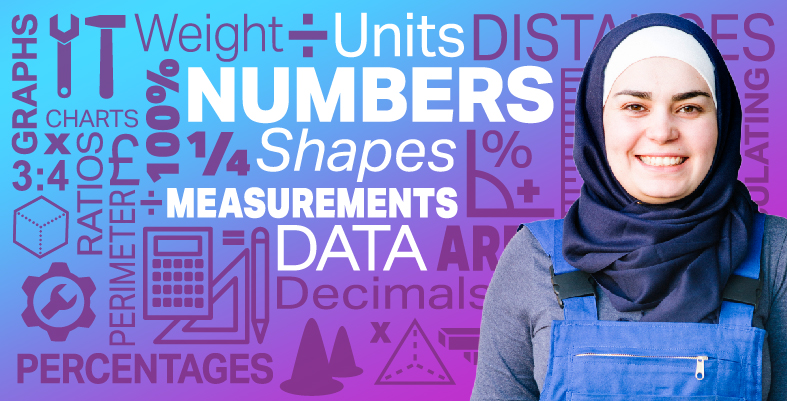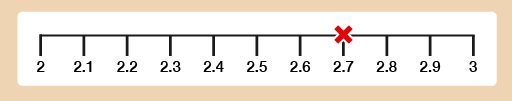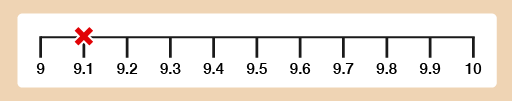Everyday maths for Construction and Engineering 1

Start this free course now. Just create an account and sign in. Enrol and complete the course for a free statement of participation or digital badge if available.

Free course

# 4.1 Approximations with decimals

Now you have looked at the place value system for decimals, can you use your rounding skills to estimate calculations using decimals? This skill would be needed in everyday life to approximate the cost of your tools and equipment for a job.

## Example: Approximations with decimals

Give approximate answers to these. Round each decimal number to the nearest whole number before you calculate.

1. 2.7 + 9.1
2. 9.6 – 2.3
3. 2.8 × 2.6
4. 9.6 × 9.5

### Method

1. 2.7 lies between 2 and 3, and is nearer to 3 than 2.Figure 17 A number line

9.1 lies between 9 and 10, and is nearer to 9 than 10.Figure 18 A number line

• 3 + 9 = 12
2. Similarly, 9.6 lies between 9 and 10 and is nearer to 10 than 9, and 2.3 is nearer to 2 than 3. So our approximate answer is:
• 10 – 2 = 8
3. 2.8 is nearer to 3 than 2, and 2.6 is also nearer to 3 than 2. So our approximate answer is:
• 3 × 3 = 9
4. 9.6 is nearer to 10 than 9. 9.5 is exactly halfway between 9 and 10. When this happens we always round up, meaning that 9.5 is rounded up to 10. So our approximate answer is:
• 10 × 10 = 100

## Example: Rounding to two decimal places

You may be asked to round a number to two decimal places. All this means is if you are faced with lots of numbers after the decimal point, you will be asked to only leave two numbers after the decimal point. This is useful when a calculator gives us lots of decimal places.

1. Round 3.426 correct to two decimal places (we want two digits after the decimal point).

### Method

Look at the third digit after the decimal point.

If it is 5 or more, round the previous digit up by 1. If it is less than 5, leave the previous digit unchanged.

The third digit after the decimal point in 3.426 is 6. This is more than 5, so you should round up the previous digit, 2, to 3.

1. Round 2.8529 to two decimal places.

### Method

As in part (a) above, the question is asking you to round to two digits after the decimal point.

Look again at the third digit after the decimal point.

This is 2 (less than 5) so we leave the previous digit (5) unchanged.

1. Round 1.685 to two decimal places.

Here, the third digit after the decimal point is 5, which means the previous digit (8) needs to be rounded up.

Hint: ‘Five or more, raise the score!’

## Activity 12: Rounding

1. Work out approximate answers to these by rounding each decimal number to the nearest whole number:
• a.3.72 + 8.4
• b.9.6 – 1.312
• c.2.8 × 3.4
• d.9.51 ÷ 1.5
2. Round the following numbers to two decimal places:
• a.3.846
• b.2.981
• c.3.475

1. The answers are as follows:
• a.The nearest whole number to 3.72 is 4.

The nearest whole number to 8.4 is 8.

4 + 8 = 12

• b.The nearest whole number to 9.6 is 10.

The nearest whole number to 1.312 is 1.

10 – 1 = 9

• c.The nearest whole number to 2.8 is 3.

The nearest whole number to 3.4 is 3.

3 × 3 = 9

• d.The nearest whole number to 9.51 is 10.

The nearest whole number to 1.5 is 2.

10 ÷ 2 = 5

2. The answers are as follows:
• a.To round to two decimal places, look at the third digit after the decimal point. This is more than 5, so round the previous digit (4) up to 5.

• b.In this case, the third digit after the decimal point is less than 5, so leave the previous digit unchanged.

• c.The third digit after the decimal point here is 5. Remember in this case we always round up.

FSM_SSC_1